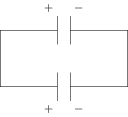# Energy Stored in Capacitor

## Problems from IIT JEE

Problem (IIT JEE 2002): Two identical capacitors have the same capacitance $C$. One of them is charged to potential $V_1$ and the other to $V_2$. The negative ends of the capacitors are connected together. When the positive ends are also connected, the decrease in energy of the combined system is,

1. $\frac{1}{4}C\left(V_{1}^{2}-V_{2}^{2}\right)$
2. $\frac{1}{4}C\left(V_{1}^{2}+V_{2}^{2}\right)$
3. $\frac{1}{4}C\left(V_{1}-V_{2}\right)^2$
4. $\frac{1}{4}C\left(V_{1}+V_{2}\right)^2$

Solution:Initially, total electrostatic energy stored in two capacitors is $U_i=\frac{1}{2}CV_1^2+\frac{1}{2}CV_2^2$. Initial charges on two capacitors are $q_1=CV_1$ and $q_2=CV_2$. Let $q_1^\prime$ and $q_2^\prime$ be charges on capacitors when same terminals of two capacitors are connected to each other (see figure). By charge conservation, \begin{alignat}{2} \label{rdb:eqn:1} &q_1^\prime+q_2^\prime=q_1+q_2. \end{alignat} The potential $V^\prime$ across two capacitors is equal, i.e., \begin{alignat}{2} \label{rdb:eqn:2} &q_1^\prime /C=q_2^\prime /C. \end{alignat} Solve above equations to get, \begin{align} &q_1^\prime=q_2^\prime=(q_1+q_2)/2, &\text{and}& &V^\prime=(V_1+V_2)/2. \nonumber \end{align} Thus, final electrostatic energy stored in the two capacitors is, \begin{alignat}{2} &U_f=\tfrac{1}{2}CV^2+\tfrac{1}{2}CV^2=\tfrac{1}{4}C(V_1^2+V_2^2+2V_1V_2), \nonumber \end{alignat} and loss in energy is $U_i-U_f=\frac{1}{4}C(V_1-V_2)^2$.